# How To Prepare for TCS NQT Quantitative Aptitude: Topics, Sample Questions And Answers

###### 28 Jul'2213 min read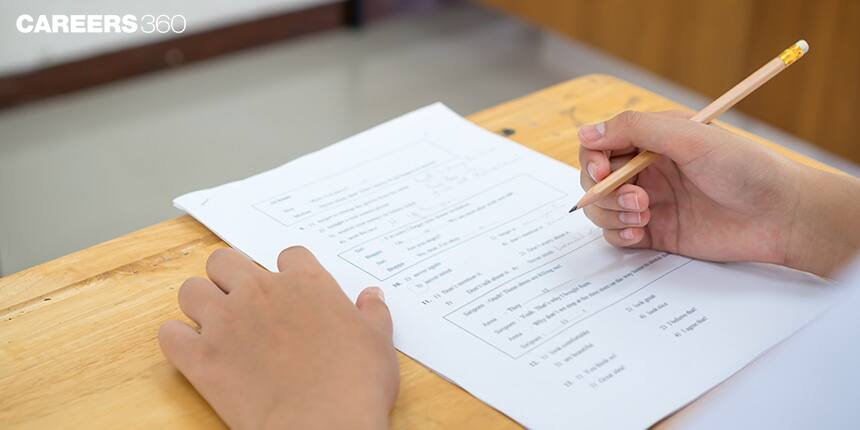###### Synopsis

Tata Consultancy Services National Qualifier Test (TCS NQT) is a recruitment test conducted by TCS, an IT services, digital and business solutions company. This article will provide you with all the information about the TCS NQT Quantitative Aptitude test. Read on to know about the topics, sample questions and answers of tcs nqt aptitude test.###### Synopsis

Tata Consultancy Services National Qualifier Test (TCS NQT) is a recruitment test conducted by TCS, an IT services, digital and business solutions company. This article will provide you with all the information about the TCS NQT Quantitative Aptitude test. Read on to know about the topics, sample questions and answers of tcs nqt aptitude test.

Tata Consultancy Services (TCS), a leading software company of India hires freshers for various profiles across India through the TCS National Qualifier Test (TCS NQT) 2022 for the 2023 batch. Registration is open on TCS’s official website: tcs.com. A national level score is issued to all the candidates and on the basis that students will be called for further process.

### Important Dates For October Exam

1. Registration: Till 24 September 2022.

2. Hall Ticket: Can be downloaded 2 days before exam.

3. Exam: Expected to start from 9 October 2022 onwards.

Note: The test will be conducted at centres allotted by TCS authorities and will be mentioned on Hall Ticket.

Also, students can download the following TCS NQT sample papers via the given links.

 Title Download Link TCS NQT Sample Paper 1 Set 1 TCS NQT Sample Paper 2 Set 2 TCS NQT Sample Paper 3 Set 3 TCS NQT Sample Paper 4 Set 4 TCS NQT Sample Paper 5 Set 5

### Eligibility Criteria

Qualification: B.E/B.Tech/M.E/M.Tech/MCA/M.Sc Students (Those who are in their final year of graduation can also apply)

Percentage Criteria: 60% or 6 CGPA in X/XII/Diploma/UG/PG

Backlogs: Only one active backlog allowed

Academic Gap: Maximum two years of gap allowed

Work Experience: Freshers or experience of up to 2 years

## TCS 2022 NQT Registration – How to Apply?

Visit the official TCS NQT registration website – www.tcs.com/careers/TCSFresherHiringYoP2023

### Exam Pattern:

TCS NQT 2022 test is expected to be in two sections as given below:

Part A- Foundation Section (ninja profile)

Part B- Advanced Section (digital profile)

 Part A (Foundation Section) Verbal Ability: This section will test your skills of reading comprehension and written communication. 25 Questions 75 minutes(Shared) Numerical Ability: This section will test your skills of deducting useful information from a given data and your numerical ability to solve the problem. 20 Questions Reasoning Ability: This section focuses on your logical and critical thinking. How efficiently will you solve everyday problems? 20 Questions Traits: Test your psychometric ability, what is your thinking? 5 Questions
 Part B (Advance Section) Advanced Quantitative Ability: - Advance level of mathematical problems will be asked in this section. 10 Questions 35 minutes(Shared) Advanced Coding: - Advance level of coding problems will be asked in this section based on your skills in Java and C language. 10 Questions Advanced Reasoning Ability: - Apply your deductive and inductive knowledge in advance level of problems which require critical thinking. 2 Questions

*No Negative Marking will be there.

### Selection Procedure:

1. Online test

2. Technical interview

3. Managerial interview

4. HR round

## Importance Of Quantitative Aptitude For TCS NQT

As we can see from the exam pattern mentioned above, the weightage of numerical ability is much greater. While preparing for NQT one should focus on the quant section. Without scoring in Numerical Ability, you will not be able to make a good score. Practising sample TCS NQT aptitude questions are important

## TCS NQT Aptitude Syllabus

For TCS NQT quantitative aptitude the following topics are to be prepared:

1. Elementary Statistics

2. Data Interpretation

3. Simplification And Approximation

4. Arithmetic: Percentage Based Questions Including Profit And Loss, Simple Interest And Compound Interest.

5. Average And Linear Equation, Application Of These Concepts In The Problems Of Ages.

6. Number System: Unit Digit, Last Two Digits, Factors, Number Of Trailing Zeroes, Remainders.

7. Ratio And Proportion, Application Of Ratio In Time And Work, Mixture And Allegations.

8. Mensuration And Geometry

9. Clock And Calendar

10. Permutations And Combination, Probability

11. Series And Progressions: Arithmetic And Geometric

12. Time, Speed And Distance

13. Function

## How To prepare Quantitative Aptitude For TCS NQT

Candidates have to plan the TCS NQT aptitude preparation by dividing the syllabus in subsections. They are advised to divide the whole syllabus into 5-6 sections such as:

1. Percentage based questions: it will cover the topics like Percentage, Profit And Loss, Simple And Compound Interest. It is advised to learn fraction to percentage conversion and vice versa to solve the problems quickly. Try to do most calculations mentally and have to practice for the same in time being.

Sample problem 1: Three companies are working independently and receiving savings of 20 %, 30 %, and 40 %. If the companies work combined, what will be their net savings?

Solution:

Suppose the amount for each company is 100

So, saving of company 1 = 20

saving of company 2 = 30

saving of company 3 = 40

Total Saving = 90

Saving % if all the three company work combined = (90 / 300) * 100 = 30 %.

Sample Problem 2: The printed price of an article was 30% less than the suggested retail price. Deeksha purchased the article for half of the marked price at the independence sale. What percent less than the suggested retail price did Deeksha pay?

a ) 60 %

b ) 20 %

c ) 65 %

d ) 30 %

Solution:

Let Suggested Retail Price be Rs. 100

Therefore, the Marked Price will be Rs. 70

Amount paid by Deeksha is 50% 0f the Marked Price = Rs. 35

So, Deeksha paid 65% less than the Suggested Retail Price. Therefore, option C is correct.

Sample Problem 3: Analysing the good returns that HDFC retail banking was giving, Srishti bought a 1 - year, Rs 20,000 certificate of deposit that paid interest at an annual rate of 12 % compounded half-annually.

What amount of interest was expected on this certificate at maturity?

Solution:

In the case of half-yearly compounding, Interest rate becomes half i.e 6 % per six months and one year has two half years.

So, interest will be 6 % of 20000 + 6 % of 20000 + 6 % of 6 % of 20000 = Rs 2472

1. Ratio based questions:

It will cover a few topics like ratio and proportion, Mixture and alligation, Time and Work, even some problems related to time, speed and distance. Try to use the concept of proportionality in Time and Distance. Problems related escalator, Circular races might get tactical so focus on those problems too.

Sample Problem 4: 4 men can build a wall in 8 days working 5 hours regularly. What are the total hours when 2 men will build the two similar walls in 20 days?

Solution:

Use the given formula

(M1 . D1 . R1) / W1 = (M2 . D2 . R2) / W2

M1 = 4, M2 = 2, W2 = 2W1, D1 = 8 days, D2 = 20 days, R1 = 5 Hours, R2 = ?

4 x 8 x 5 / W1 = 4 x 20 x R2 / 2W1

On solving, R2 = 8 Hrs.

Sample Problem 5: The savings are calculated as the difference of income and expenditure. If the income of P, Q, and R are in the ratio 10:12:13 and their expense ratio 31:22:13 then what is the order of the P, Q, and R in increasing order of the size of their savings?

a ) P > Q > R

b ) P < Q < R

c ) P < R < Q

d ) P > R > Q

Solution:

For P, Income is the least, and expenditure is the most, therefore saving will be the least for P.

For R, Income is Maximum, and expenditure is least, therefore saving will be maximum for R.

Therefore, option b) P < Q < R shows the correct sequence.

Sample Problem 6: Two trains Marudhar Express and Vandebharat Express running in opposite directions cross a man standing on the platform in 37 seconds and 23 seconds respectively. If they cross each other in 33 seconds, what is the ratio of their speeds?

Solution:

Let the speed of the trains be x and y respectively

length of train1 = 37 x

length of train2 = 23 y

Relative speed= x + y

Time taken to cross each other = 33 s

=> (37 x + 23 y) / (x + y) = 33

=> (37 x + 23 y) = 33 (x + y)

=> 4 x = 10 y

=> x / y = 10 / 4 = 5 / 2

1. Mensuration and Geometry

Learn all the formulas with the basics. Learn the difference between curved surface area and Total Surface area as students make mistakes in this. Practice more and more questions on this. Problems based on the application of mensuration in real life have been asked previously.

We have various interesting problems in geometry related to angles formed at the centre of a circle, Different kinds of triangles, point of intersection of median, altitude, perpendicular bisectors, angle bisectors and what that point be called? How does the exterior angle of a polynomial relate to interior angles of a polynomial? The shapes to be asked might be a combination of triangles, quadrilateral, circles. It is never going to be easy. Such topics to be covered in detail. This section is quite helpful in solving TCS NQT aptitude questions.

Sample Problem 7: Two vertical rods of length of 16 m and 12 m are kept vertically and their feet are 3m apart. Find the top distance of both rods?
Sol: Distance between their heights = 16 - 12 = 4 m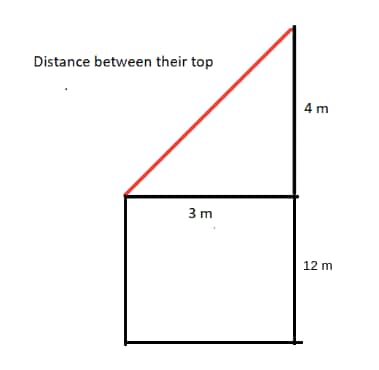Using Pythagoras theorem, the Distance between their top points = √ ( 42 + 32 ) = 5 m

Sample Problem 8: A rectangle is divided into four rectangles with area 70, 36, 20, and x. The value of x is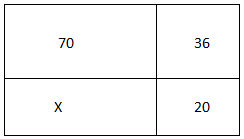a ) 350 / 9

b ) 350 / 7

c ) 350 / 11

d ) 350 / 13

Solution: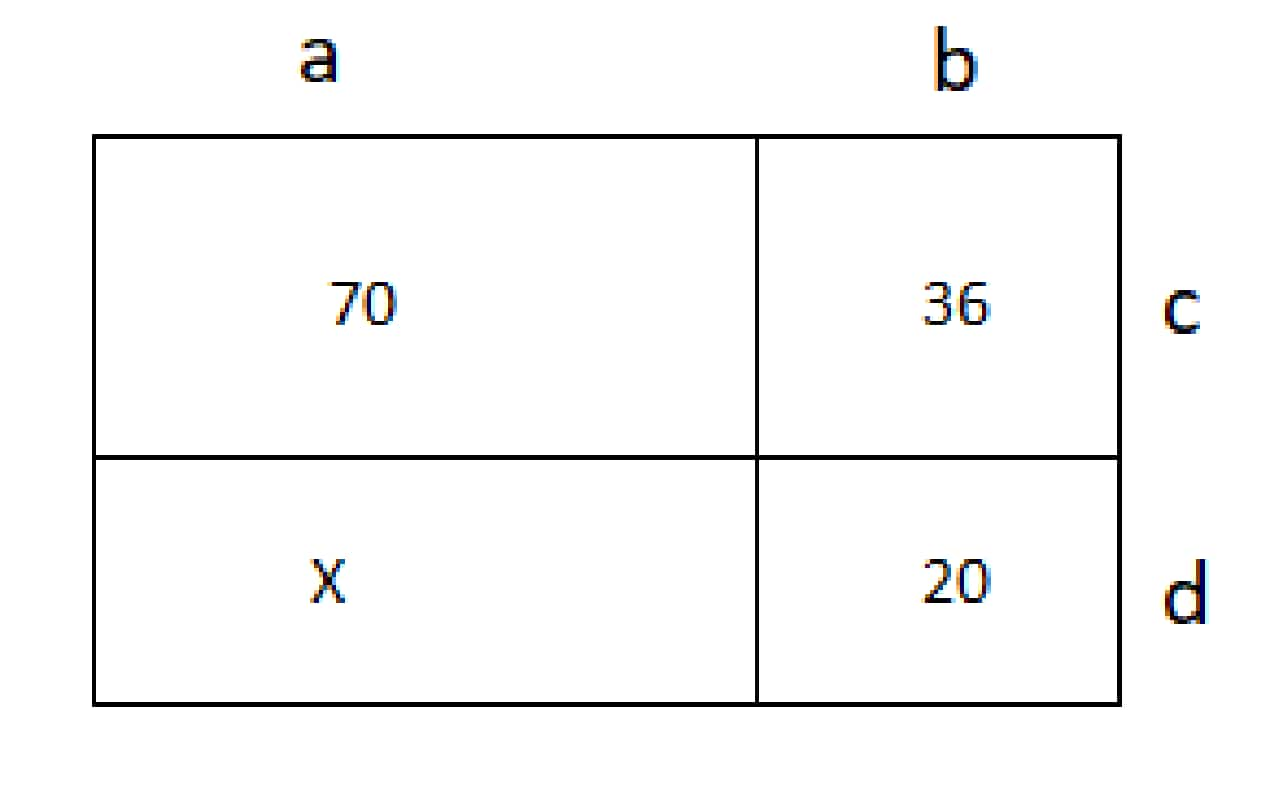Sides of the rectangle are assumed as shown in figure.

Area of rectangle = length x breadth

Therefore, from above figure,

a x c = 70,

b x c = 36

b x d = 20

so, a x d = (70 x 20 / 36) = 350 / 9, therefore option a) 350 / 9 is correct.

1. Permutation and Combination along with Probability

Questions on basic principle of counting, linear arrangement, circular arrangement are important from this topic. To solve these questions, one should have a clear understanding of permutation and combination. If you have to find a number of ways of selecting 2 objects out of 5, which concept has to be used, permutation or combination? Answer is a combination to be used here.

Problems on probability are important for the TCS NQT exam. For example, let’s say a student appears in the 12th board exam, 5 subjects are there, what is the probability that he failed in more than two subjects? Having an explicit understanding of the different approaches of solving probability problems will provide an edge to your preparation for the TCS NQT exam. Simple problems of probability may also combine with different situations to make problems complicated. An idea about using the concept of probability in different scenarios will certainly help you. With the help of permutations and combinations, most of the problems on probability can be easily solved. Continuous practice of various quantitative aptitude questions will help in accelerating preparation for NQT.

Sample Problem 9: There are 4 balls and 4 boxes of colors yellow, pink, red, and green.

Red ball is in a box with the color of the ball in the yellow box.

Red box contains a green ball.

In which box you find the yellow ball?

Solution:

Yellow box cannot have a green ball since it is in the red box.

Yellow box can not have a red ball, if it does so, the red ball should be in the red box which is not possible since one ball can not be in two boxes.

Therefore, Yellow box can have either a pink or yellow ball. If we put a yellow ball in the "yellow" box then it would imply that "yellow" is also the color of the box which has the red ball which is not possible.

Thus this possibility is ruled out.

Therefore the yellow box must have a pink ball, hence the color of the box in which the red ball is there, is pink.

Therefore the yellow ball must be in the green box.

1. Number System

Learn the basic concept of unit digit like what comes from the unit place of 57n? How many trailing zeros are there in 32!? And more questions.

To solve such problems, we need to learn the concepts related to finding the last two digits of a number, factor theorem, remainder theorem, etc.

Sometimes problems can be solved by assuming values (Hit and Trail). HCF and LCM are very important.

Sample Problem 10:

X = 201202203204205206207......246247248249250 (From numbers 201-250). What will be the remainder when the given number is divided by 3.

Solution:

As we know if the sum of digits of a number is divisible by 3 then the number itself is divisible by 3.

therefore, in this process we will find:

Sum of the digits in hundreds place (a)= 2 x 50 = 100

Sum of the digits in tenths place (b) = 0 x 9 + 1 x 10 + 2 x 10 + 3 x 10 + 4 x 10 + 5 x 1 = 105

Sum of the digits in unit’s place (c) = (1 + 2 + 3 + ...+ 9) x 5 = 225

So, total (d) = a + b + c = 100 + 105 + 225 = 430

So, to find the remainder take the sum of digits of total (d) = 4 + 3 + 0= 7

If we divide 7 by 3 we will get the remainder as 1. Therefore the answer is 1.

Sample Problem 11:

91 + 92 + 93 + ....... + 9105. Find out how many terms in this sum contain 1 at their unit place?

Solution:

Unit digits of the first 2 terms are 9 and 1. and it will go on repeating till last term. Therefore, for every 2 terms we get one term ending with 1 in its unit digit. So, there are a total of 105/2 = 52 sets and each set contains one term with 1 in its unit digit.

Sample Problem 12:

( p / q - q / p) = 21 / 10. Then find 4 p / q + 4q / p?

Solution:

Let p / q = a, then ( a - 1 / a ) = 21 / 10

So, 4p / q + 4q / p = 4( a + 1 / a)

( a + 1 / a)2 = ( a - 1 / a)2 + 4 = ( 21 / 10 )2 + 4 = 841 / 100

So, ( a + 1 / a) = ± 29 / 10

Therefore, ( 4p / q + 4q / p ) = 4( a + 1 / a ) = ± 58 / 5

1. Miscellaneous

All the remaining topics like Average, function, Series, Logarithm grouped while preparing for TCS NQT.

Sample Problem 13:

The sticks of equal lengths are used to form a triangle (equilateral triangle) as shown in figure. If a student uses 137 such sticks, then how many triangles will he be able to form?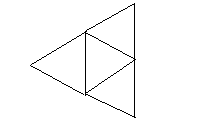a ) 68

b ) 62

c ) 65

d ) 69

Solution:

Three sticks make one triangle. Two more sticks are required to form one more triangle and this process will continue.

Therefore, we can conclude that 5 sticks are required for two triangles, 7 required for 3 triangles and so on…….

So, the number of triangles formed will be 68.

Sample Problem 14:

While entering a fun park, here are the rate list as given below:

Entry fee is Rs.10

Charges for 3 rides = Rs. 5 per ride

A total of 3000 boys entered the fun park. Out of them 800 took all the three rides, 1400 went for at least two rides and none of them went for the same ride twice. If the total expenses in the fun park was Rs. 51000.

Then how many students did not take any ride?

a ) 1800

b ) 4200

c ) 1000

d ) None of these

Solution:

Total Entry fee = Rs 10 x 3000 = Rs 30000

Total Expenses for 800 students (for 3 rides) = Rs. 15 x 800 = Rs. 12000

Total Expenses for 600 (1400-800) students for 2 rides = Rs 10 x 600 = Rs 6000

Expenses for students who went for only one ride = Rs 51000- (Rs. 30000 + Rs 12000 + Rs 6000 = Rs 3000)

Number of students who went for only one ride = 3000/5 = 600

Number of students who did not went for no ride = 3000 - 800 - 600 - 600 = 1000

Sample Problem 15:

In 4 years, Ashok's father will be twice old as Ashok. Two years ago, Ashok's mother's age was two times that of Ashok. If Ashok’s age will be 32 yrs after eight years from now, what is the age of Áshok’s parents?

Solution:

Ashok’s present age= 32 - 8 = 24.

After 4 years:

Ashok’s age= 24 + 4 = 28

Ashok's father’s age = 28 x 2 = 56.

Ashok's father’s present age = 56 - 4 = 52.

Two years ago:

Ashok's age= 24 - 2 = 22

Ashok's mother's age is 22 x 2 = 44.

His mother's present age = 46

Sample Problem 16:

In a series of numbers, the next term is formed by adding 5 to the sum of the previous terms, and the 8th term is 960. Then what is the first term of the series?

a ) 10

b ) 14

c ) 15

d) None of these

e) Can not be determined

Solution:

The given series can be written as x, x + 5, 2 x + 10, 4 x + 20, …………..

If we see the pattern, the eighth term will be 64 x + 40.

Therefore 64 x + 320 = 960,

On solving, we get x = 10.

Sample Problem 17:

The number of multiples of 6 which are less than 100, which can be written as a sum of three consecutive integers are

a ) 49

b ) 50

c ) 16

d ) 97

Solution:

Let the three integers be a, a + 1, a + 2. So

a + ( a + 1) + ( a + 2 ) = 6n, where n is a positive integer.

3a + 3 = 6n

a + 1 = 2n

a = 2n -1

So values of a can be 1, 3, 5, 7, ………… 31 (check by putting values of n so that a, a+1, and a+2 must be less than 100) is an integer for k = an odd number.

So there are 16 numbers.

Sample Problem 18:

Mr. Pushpa chooses a number, doubles it and subtracts 1 from the result. if he started with 5 as the initial number and repeats the operation for 33 times then what is the final number?

a ) 233 + 1

b ) 233 + 2

c ) 235 – 1

d ) 235 + 1

Solution:

Step 1: ( 5 x 2 ) - 1 = 9 = ( 23 + 1 )

Step 2: ( 9 x 2 ) - 1 = 17 = ( 24 + 1 )

Step 3: ( 17 x 2 ) - 1 = 33 = ( 25 + 1 )

So After 33 steps, we have 235 + 1

• Geometry
• Mathematics
• Interest
• Elementary mathematics
• Area
• Triangle
• Bisection
• Altitude (triangle)
• Angle
• Circle

## Subscribe toMembership Plan

##### *Unlock all premium content and benefits:
Read more from Latest Stories

## Careers360 helping shape your Career for a better tomorrow#### 250M+

Students#### 30,000+

Colleges#### 500+

ExamsE-Books